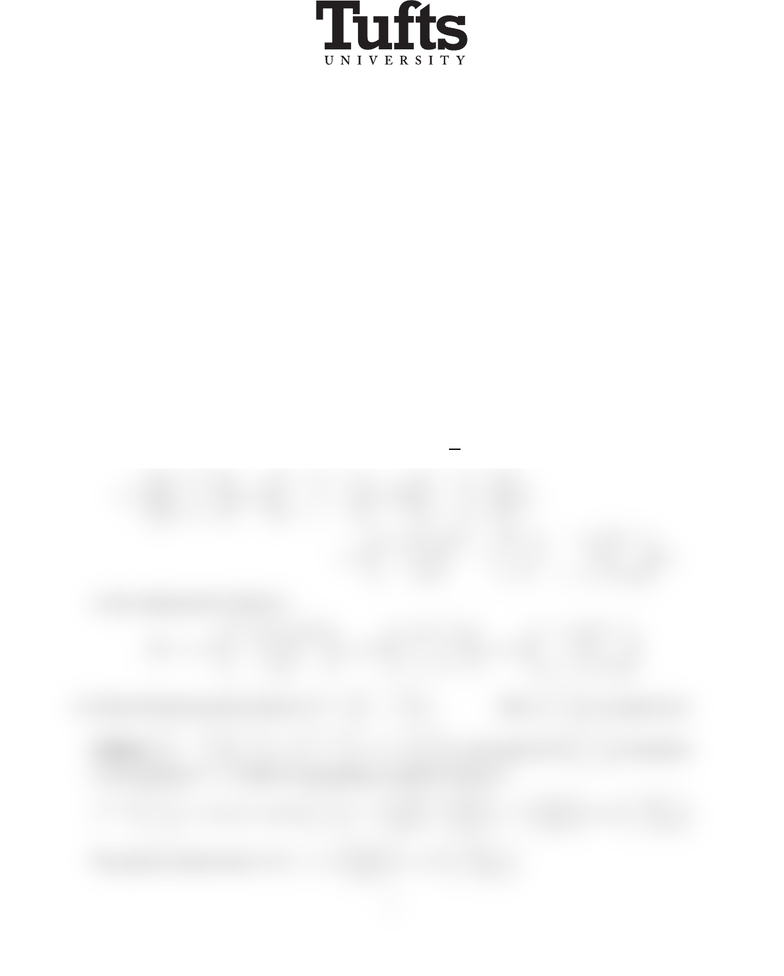# MATH51 PastExams Sol3-F11

20 views2 pages
School
Tufts University
Department
Mathematics
Course
MATH-0051
ProfessorMathematics 38 Differential Equations
Exam III December 5, 2011
No calculators, books or notes are allowed on the exam. All electronic devices must be turned off
and put away.You must show all your work in the blue book in order to receive full credit. Please
book. With your signature you are pledging that you have neither given nor received assistance on
the exam.
Good luck!
1. (10 points) Given the matrix A=13
3 1 and the eigenvector ~v =1
1ﬁnd
a. the eigenvalue λof Ato which ~v corresponds and
b. the associated solution of D~x =A~x.
Solution: a. 13
3 1 1
1=2
2=21
1so λ=2.b. e2t1
1.
2. (20 points) The 3×3matrix A=
33 1
100
010
has a triple eigenvalue of 1(you do not have to
verify this). Find the general solution of
D~x =A~x.
Solution: ~v1=
1
0
0
,~v2=
0
1
0
,~v3=
0
0
1
are (obviously!!) generalized eigenvectors.
Each of these gives a solution hi(t) = et[I+t(AI) + t2
2(AI)2]~vi=
=et
1 0 0
0 1 0
0 0 1
+t
23 1
11 0
0 1 1
+t2
2
12 1
12 1
12 1
~vi
=et
1 + 2t+t2/23tt2t+t2/2
t+t2/2 1 tt2t2/2
t2/2tt21t+t2/2
~vi,
so the sought general solution is
~x(t) = c1et
1 + 2t+t2/2
t+t2/2
t2/2
+c2et
3tt2
1tt2
tt2
+c3et
t+t2/2
t2/2
1t+t2/2
3. (20 pts) Find the general solution of ~x=11
1 1 ~x. Hint: 1
iis an eigenvector.
Solution: 11
1 1 1
i=1 + i
= (1+i)1
, so the eigenvector 1
icorresponds
to the eigenvalue 1 + i, and the corresponding (complex) solution is
e(1+i)t1
i=et(cos t+isin t)1
i=etcos t+isin t
sin ticos t=etcos t
sin t+ietsin t
cos t.
The general solution then is ~x(t) = c1etcos t
sin t+c2etsin t
cos t.
1
Unlock document

This preview shows half of the first page of the document.
Unlock all 2 pages and 3 million more documents.

## Document Summary

No calculators, books or notes are allowed on the exam. All electronic devices must be turned off and put away. you must show all your work in the blue book in order to receive full credit. Please box your answers and cross out any work you do not want graded. With your signature you are pledging that you have neither given nor received assistance on the exam. Good luck: (10 points) given the matrix a = (cid:18) 1 3. 1(cid:19) and the eigenvector ~v = (cid:18) 1. 3 a . the eigenvalue of a to which ~v corresponds and: the associated solution of d~x = a~x. Solution: a. (cid:18) 1 3: (20 points) the 3 3 matrix a = . 1(cid:19) so = 2. b. e 2t(cid:18) 1. Has a triple eigenvalue of 1 (you do not have to. 1 2 so the sought general solution is. Each of these gives a solution hi(t) = et[i + t(a i) + t.